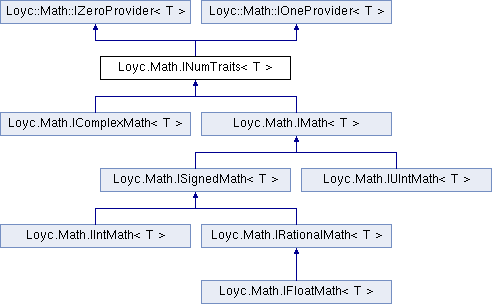Enhanced C# Language of your choice: library documentation
Loyc.Math.INumTraits< T > Interface Template Reference

This interface provides information about a numeric type T. More...

Source file:
Inheritance diagram for Loyc.Math.INumTraits< T >:## Remarks

This interface provides information about a numeric type T.

Template Parameters
 T A numeric type

## Properties

MinValue` [get]`
Minimum value of this type above negative infinity. More...

MaxValue` [get]`
Maximum value of this type below infinity. More...

Epsilon` [get]`
Smallest representable positive value of T (1 for integer types). More...

PositiveInfinity` [get]`
Returns positive infinity, or MaxValue for types that cannot represent infinity. More...

NegativeInfinity` [get]`
Returns negative infinity, or throws NotSupportedException if T is unsigned. More...

NaN` [get]`
Not-a-number or null representation for this type. More...

bool IsSigned` [get]`
Returns true if T can represent negative values. More...

bool IsFloatingPoint` [get]`
Returns true if T is floating-point, meaning that it can represent very large and very small numbers, despite possibly limited precision. Returns false for fixed-point and integer-rational types. More...

bool IsInteger` [get]`
Returns true if the type represents only whole numbers. More...

bool IsOrdered` [get]`
Returns true for "normal" numbers, false for ones that aren't necessarily comparable (notably complex numbers). More...

int SignificantBits` [get]`
Returns the normal maximum number of significant (mantissa) bits for this type (not counting the sign bit), or int.MaxValue for unlimited-size types. More...

int MaxIntPowerOf2` [get]`
Returns the maximum power-of-two-minus-one that can be represented by this type, e.g. for Int32 it's 31, and for UInt32 it's 32. More...

ulong MaxInt` [get]`
Returns the maximum integer that this type can represent. More...

long MinInt` [get]`
Returns the minimum integer that this type can represent. More...Properties inherited from Loyc.Math.IZeroProvider< T >
Zero` [get]`
Returns the "zero" or additive identity of this type. More...Properties inherited from Loyc.Math.IOneProvider< T >
One` [get]`
Returns the "one" or identity value of this type. More...

## Public Member Functions

bool IsInfinity (T value)
Returns true if the given value is infinite. More...

bool IsNaN (T value)
Returns true if the given value is not a number (can only be true for floats). More...

Floor (T value)
Gets the closest integer equal to or lower than the specified number. More...

Ceiling (T value)
Gets the closest integer equal to or higher than the specified number. More...

## ◆ Ceiling()

 T Loyc.Math.INumTraits< T >.Ceiling ( T value )

Gets the closest integer equal to or higher than the specified number.

For integer types, this has no effect.

## ◆ Floor()

 T Loyc.Math.INumTraits< T >.Floor ( T value )

Gets the closest integer equal to or lower than the specified number.

For integer types, this has no effect.

## ◆ IsInfinity()

 bool Loyc.Math.INumTraits< T >.IsInfinity ( T value )

Returns true if the given value is infinite.

Types that do not have an infinity value always return false from this method.

## ◆ IsNaN()

 bool Loyc.Math.INumTraits< T >.IsNaN ( T value )

Returns true if the given value is not a number (can only be true for floats).

## ◆ Epsilon

 T Loyc.Math.INumTraits< T >.Epsilon
get

Smallest representable positive value of T (1 for integer types).

## ◆ IsFloatingPoint

 bool Loyc.Math.INumTraits< T >.IsFloatingPoint
get

Returns true if T is floating-point, meaning that it can represent very large and very small numbers, despite possibly limited precision. Returns false for fixed-point and integer-rational types.

## ◆ IsInteger

 bool Loyc.Math.INumTraits< T >.IsInteger
get

Returns true if the type represents only whole numbers.

## ◆ IsOrdered

 bool Loyc.Math.INumTraits< T >.IsOrdered
get

Returns true for "normal" numbers, false for ones that aren't necessarily comparable (notably complex numbers).

## ◆ IsSigned

 bool Loyc.Math.INumTraits< T >.IsSigned
get

Returns true if T can represent negative values.

## ◆ MaxInt

 ulong Loyc.Math.INumTraits< T >.MaxInt
get

Returns the maximum integer that this type can represent.

If the maximum integer exceeds ulong.MaxValue, this returns ulong.MaxValue.

## ◆ MaxIntPowerOf2

 int Loyc.Math.INumTraits< T >.MaxIntPowerOf2
get

Returns the maximum power-of-two-minus-one that can be represented by this type, e.g. for Int32 it's 31, and for UInt32 it's 32.

## ◆ MaxValue

 T Loyc.Math.INumTraits< T >.MaxValue
get

Maximum value of this type below infinity.

## ◆ MinInt

 long Loyc.Math.INumTraits< T >.MinInt
get

Returns the minimum integer that this type can represent.

If the minimum is less than long.MinValue, this returns long.MinValue.

## ◆ MinValue

 T Loyc.Math.INumTraits< T >.MinValue
get

Minimum value of this type above negative infinity.

## ◆ NaN

 T Loyc.Math.INumTraits< T >.NaN
get

Not-a-number or null representation for this type.

Exceptions
 NotSupportedException There is no null or NaN value for type T.

## ◆ NegativeInfinity

 T Loyc.Math.INumTraits< T >.NegativeInfinity
get

Returns negative infinity, or throws NotSupportedException if T is unsigned.

Exceptions
 NotSupportedException T is unsigned.

## ◆ PositiveInfinity

 T Loyc.Math.INumTraits< T >.PositiveInfinity
get

Returns positive infinity, or MaxValue for types that cannot represent infinity.

## ◆ SignificantBits

 int Loyc.Math.INumTraits< T >.SignificantBits
get

Returns the normal maximum number of significant (mantissa) bits for this type (not counting the sign bit), or int.MaxValue for unlimited-size types.